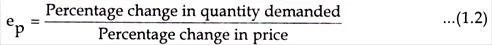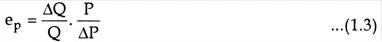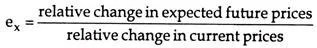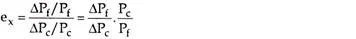# Measuring Elasticity of Demand

7th February 2020

A change in the price of a commodity affects its demand. We can find the elasticity of demand, or the degree of responsiveness of demand by comparing the percentage price changes with the quantities demanded. In this article, we will look at the concept of elasticity of demand and take a quick look at its various types.

### Elasticity of Demand

To begin with, let’s look at the definition of the elasticity of demand: “Elasticity of demand is the responsiveness of the quantity demanded of a commodity to changes in one of the variables on which demand depends. In other words, it is the percentage change in quantity demanded divided by the percentage in one of the variables on which demand depends.”

The following points highlight the top five methods used for measuring the elasticity of demand. The methods are:

1. Price Elasticity of Demand
2. Income Elasticity of Demand
3. Cross Elasticity of Demand
5. Elasticity of Price Expectations.

Method 1. Price Elasticity of Demand

Price elasticity of demand is a measure of the responsiveness of demand to changes in the commodity’s own price. It is the ratio of the relative change in a dependent variable (quantity demanded) to the relative change in an independent variable (Price). In other words, price elasticity is the ratio of a relative change in quantity demanded to a relative change in price.Also, elasticity is the percentage change in quantity demanded divided by the percentage in price.Symbolically, we may rewrite the formula:

If percentages are known, the numerical value of elasticity can be calculated. The coefficient of elasticity of demand is a pure number i.e. it stands by itself, being independent of units of measurement. The coefficient of price elasticity of demand can be calculated with the help of the following formula.Where,

Q is quantity, P is price, ΔQ/Q relative change in the quantity demanded and ΔP/P Relative change in price.

It should be noted that a minus sign (-) is generally inserted in the formula before the fraction with a view to making the coefficient of elasticity a non-negative value.

The price elasticity can be measured between two finite points on a demand curve (called arc elasticity) or on a point (called point elasticity).

Method 2. Income Elasticity of Demand

The responsiveness of quantity demanded to changes in income is called income elasticity of demand. With income elasticity, consumer incomes vary while tastes, the commodity’s own price, and the other prices are held constant.

The income elasticity of demand for a good or service may be calculated by the formula:where- ey stands for the coefficient of income elasticity, Y for income.

Whereas price-elasticity of demand is always negative, income-elasticity of demand is always positive (except for inferior goods) as the relationship between income and quantity demanded of a product is positive. For inferior goods the income elasticity of demand is negative because as income increases, consumers switch over to the consumption of superior substitutes.

Method 3. Cross Elasticity of Demand

Demand is also influenced by prices of other goods and services. The cross elasticity measures the responsiveness of quantity demanded to changes in price of other goods and services. Cross elasticity of demand is defined as the percentage change in quantity demanded of one good caused by a 1 percentage change in the price of some other good.Cross elasticity is used to classify the relationship between goods. If cross elasticity is greater than zero, an increase in the price of y causes an increase in the quantity demanded of x, and the two products are said to be substitutes. When the cross- elasticity is greater than zero, the goods or services involved are classified as complements Increases in the price of y reduces the quantity demanded of that product. Diminished demand for y causes a reduced demand for x. Bread and butter, cars and tires, and computers and computer programs are examples of pairs of goods that are complements.

The coefficient is positive if A and B are substitutes because the price change and the quantity change are in the same direction. The coefficient is negative if A and B are complements, because changes in the price of one commodity cause opposite changes in the quantity demanded of the other. Other things such as consumer taste for both commodities, consumer incomes and the price of the other commodity are held constant.

The advertisement expenditure helps in promoting sales. The impact of advertisement on sales is not uniform at all level of total sales. The concept of advertising elasticity is significant in determining the optimum level of advertisement outlay particularly in view of competitive advertising by rival firms. An advertising elasticity could be defined as the percentage change in quantity demanded for a percentage change in advertising. Advertising might be measured by expenditure.

Advertising elasticity may be measured by the following formula:Method 5. Elasticity of Price Expectations

People’s price expectations also play a significant role as a determinant of demand. J.R. Hicks, the English economist, in 1939, devised the concept of elasticity of price expectations. The elasticity of price expectations may be defined as the ratio of the relative change in expected future prices to the relative change in current prices.